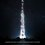# Die Hard - Algebra Geometry Problem

One Of the toughest Question Of Maths and Geometry and algebra. Can you solve?

Now, to solve this question, you have to solve some parts individually. PART(A): If we have a circle of radius R and its inscribed triangle XYZ that is acute as well as scalene. XY is the longest side.XA,YB, ZC are the altitudes of the triangle XYZ. Let,L be the symmetric point of A w.r.t. BC and M be the symmetric point of B in AC.P is the intersection of XL and YM. H is the orthocenter of triangle XYZ. Then OP.OH = f(R). You need the function f from this question to solve 'THE QUESTION'. Note: Symmetric Point of Q w.r.t. to line EW is the point that is reflection of Q in line EW.

Part(B): {bn} is a real number sequence that depends on {an} in the following way: b=a and for k ≥ 1,b[k+1] = a[k+1]-√c, where c = Σ ( a[i]a[i] ) ; i={1, 2, 3...k}. Let G be the smallest number ≥ 0 such that for all real number sequences {a_n} and all positive integers x: (1/x) Σ ( a[i]a[i] ) ≤ Σ ( pow(G,x-j)b[j]*b[j] ); i,j={1, 2, 3...x} You need G from this question to solve 'THE QUESTION'.

Part(C): Let Q be the least possible value of XY if X, Y, Z are three non-collinear points that are planar, and all of the three points have integral coordinates and all the three lengths XY, YZ, ZX have integral lengths.You need Q from this question to solve 'THE QUESTION'.

'THE QUESTION' : Let A={1,2...m} and let x belong to the set A. Then let P( that depends on m and x) be the number of functions f from A on itself, such that for every a belonging to the set A,there is a k≥0 such that fk(a) ≤ x.Note that fk is the function that is obtained by composition of the function f, k number of times i,e. f_2(x) is the function f(f(x)). Now, you need to print P(m,x)/f(m) where f is the function obtained in Part A. Put x as the value pow(G, Q) and m as the (pow(Q, pow(Q-1, G-1)) + pow(G, 2)), where G is obtained from Part B and Q is obtained from Part C.Note that you need to print the greatest integer less than or equal to the actual exact answer.Note by Parv Parkhiya
6 years, 9 months ago

This discussion board is a place to discuss our Daily Challenges and the math and science related to those challenges. Explanations are more than just a solution — they should explain the steps and thinking strategies that you used to obtain the solution. Comments should further the discussion of math and science.

When posting on Brilliant:

• Use the emojis to react to an explanation, whether you're congratulating a job well done , or just really confused .
• Ask specific questions about the challenge or the steps in somebody's explanation. Well-posed questions can add a lot to the discussion, but posting "I don't understand!" doesn't help anyone.
• Try to contribute something new to the discussion, whether it is an extension, generalization or other idea related to the challenge.

MarkdownAppears as
*italics* or _italics_ italics
**bold** or __bold__ bold
- bulleted- list
• bulleted
• list
1. numbered2. list
1. numbered
2. list
Note: you must add a full line of space before and after lists for them to show up correctly
paragraph 1paragraph 2

paragraph 1

paragraph 2

[example link](https://brilliant.org)example link
> This is a quote
This is a quote
    # I indented these lines
# 4 spaces, and now they show
# up as a code block.

print "hello world"
# I indented these lines
# 4 spaces, and now they show
# up as a code block.

print "hello world"
MathAppears as
Remember to wrap math in $$ ... $$ or $ ... $ to ensure proper formatting.
2 \times 3 $2 \times 3$
2^{34} $2^{34}$
a_{i-1} $a_{i-1}$
\frac{2}{3} $\frac{2}{3}$
\sqrt{2} $\sqrt{2}$
\sum_{i=1}^3 $\sum_{i=1}^3$
\sin \theta $\sin \theta$
\boxed{123} $\boxed{123}$